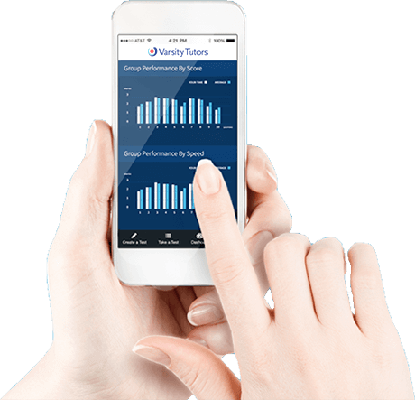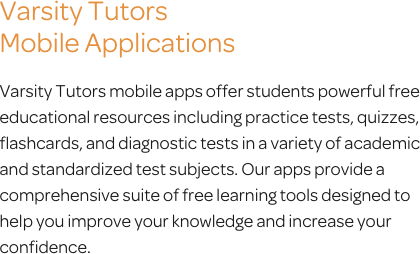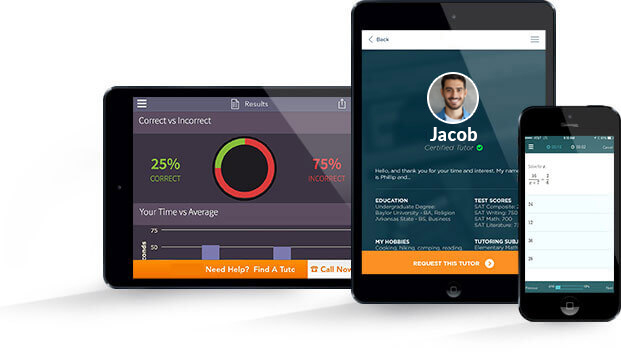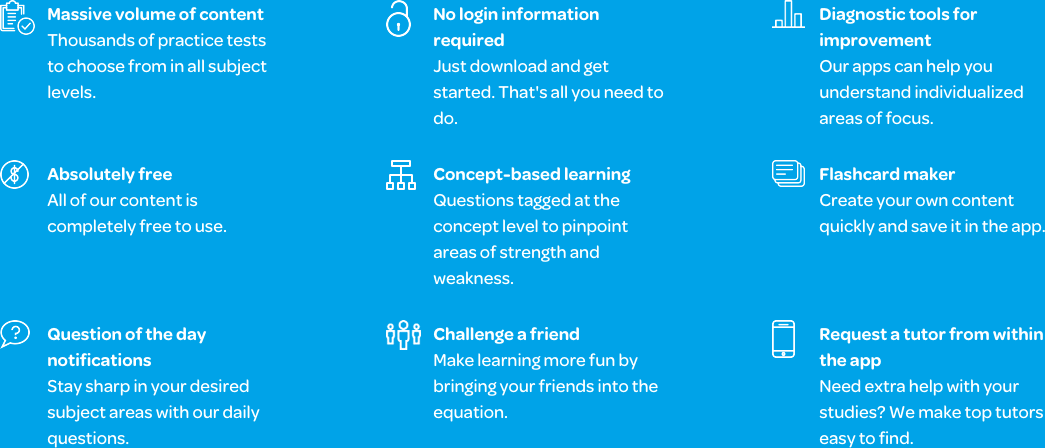# Varsity Tutors Algebra mobile app### The Varsity Tutors Algebra Mobile App

Algebra 1 is a foundational course that lays the groundwork for proficiency in future math courses. Students learn how to work with percentages, graphs, variables, and systems of inequalities. Some concepts can be challenging, but can be mastered with some practice. If your student needs a little extra help, the free Varsity Tutors Algebra 1 app for Android, iPhone, and iPad can be downloaded from iTunes or the Google Play Store today.

Diving into algebra all at once can be overwhelming, but the app lets students work at their own pace. It enables your student to focus on one concept at a time. The app can be used as a classroom supplement or exam review tool. The practice questions and full-length practice tests can help identify strengths and weaknesses. Subject flashcards also make the process easier. Your student may, for example, be learning standard deviation; related flashcards and practice questions enable them to work with relevant subject matter and reinforce essential skills.

There are hundreds of practice tests accessible from the app. With the results from the full-length practice tests, your child can review their scores and see where they need additional support. The correct answers are also explained. Your child can integrate these into their study plan, so the app can be an active part of the educational process.

Your child will encounter topics from Algebra I throughout their school years. They’ll need to know these concepts to pass their classes and advance to higher levels in math. Both state exams and many other standardized tests include algebra to assess the abilities of students. It is also part of the Common Core standards, so your child should have access to as many tools as possible to help them overcome any challenges they may encounter.

As a language of patterns, algebra is a gateway to understanding more advanced mathematics. Equations, expressions, functions, and more are vital concepts to learn. They are basic elements of this language that students need to know before they can become proficient in more complex forms of math. With the right tools, your child can practice working with exponents, ratios, and quadratic equations.

In Algebra I, your child will only need to work with one variable. Later on, the number of variables will increase. It’s essential for your child to master working with single-variable equations and practicing how to convert data between graphs and equations now. For example, a line on a graph between one point and another has an equation. Students are required to find the equation, or use the equation provided to show what it would represent on the graph.

From parabolas to FOIL, the concepts are many. Probability, statistics, and percent are other topics that may come up. The subject matter will often refer back to graphs and functions. Students must also learn how to work with logic at this level. There are many options for helping your child discover which concepts they need to spend more time practicing.

Your child might only need a quick review, but the app is also useful for continual studying and preparation. They can work at their own pace, with the guidance and support needed to master the material. All of the materials and resources have been created by professionals. The free Varsity Tutors Algebra 1 app that’s available on iTunes and the Google Play Store may be just what your child needs to address any challenges in the subject.Algebra 1 is an important foundation course for upper-level mathematics. This course is generally taken between the 7th and 12th grades, but you may encounter algebraic thinking in real-life situations well before you formally take the class. Algebra 1 is an introduction to basic algebraic concepts, in which you will learn many new problem-solving skills in number operations, lines and functions, linear equations and inequalities, statistics and probability, and variables. Proficiency in the basic skills learned in Algebra 1 is essential to future success in higher-level math and science courses, like geometry, calculus, chemistry, and physics.

To succeed in Algebra 1, you will need to have a solid mastery of basic arithmetic skills, including addition, subtraction, multiplication, division, negative and positive numbers, fractions, factors, and exponents. You will need to learn to think logically about operations and be diligent about completing each step in the solving process. You will also need to achieve proficiency in the vocabulary of algebra, as you will need to explain relationships and describe the data presented by charts, graphs, and tables.

The first concept you will learn in Algebra 1 is how to use the distributive property. You will learn to use the FOIL (first, outer, inner, last) technique to expand equations. Then, you will begin working on the skills you’ll need to successfully solve equations. There are many different types of algebraic equations, and Algebra 1 will introduce you to the fundamentals of linear, rational, variable, and quadratic equations. You’ll learn to write equations and expressions and solve one- and two-step equations. You will also find solution sets for both a single equation and a system of equations. Finally, you will begin to work with the systems of inequalities. You’ll learn to write and graph inequalities and find solutions using addition, subtraction, multiplication, and division.

Algebra 1 also covers a wide variety of concepts in number operations, particularly those dealing with percentages and integers. You will learn to find fractional percentages and convert both decimals and fractions to and from percentages. You’ll also learn to work with monetary percentage concepts like finding the amount of profit, simple interest, the amount of sales tax, and an item’s sale price. Other percent calculations covered in Algebra 1 include finding the percent of change and using percentage to find the part from a whole, or the whole from a part.

You will also be introduced to several concepts using real numbers. Counting and sets will be reviewed, and you will learn to both find a set’s missing number and to calculate the number of integers that fall between two others. Integer operations are thoroughly covered as well, and you will be taught to add, subtract, multiply, and divide with integers. The number operations unit wraps up with lessons on absolute value and number lines, like finding absolute value and using a number line to find value, graph an inequality, and plot fractions.

Next, you’ll cover functions and lines. The functions covered in Algebra 1 are essential to master, as they are widely used throughout the course in different capacities. You’ll need to know how to find f(x), direct and inverse variation, a function’s domain, and the quadratic function. You will then begin work on concepts dealing with equations of lines, like the midpoint formula, finding the equation and slope of parallel and perpendicular lines, points and distance formula, and slope and line equations. You’ll work on mathematical sequencing concepts as well, like learning to find the next through the nth terms of arithmetic sequences, consecutive integers, and the common differences in sequences.

After you have a good grasp on functions and lines, you will be introduced to graphing. Graphing is a versatile skill that you will use in a variety of classes in addition to Algebra 1. You will first learn the lingo that accompanies algebraic graphing; you’ll need to understand what the problems are asking in order to solve them. You’ll learn terms like origin, quadrant, axis, and slope intercept. Then, you will begin working on the basics: graphing functions, lines, and points. You’ll then move on to graphing quadratic functions, two-step inequalities, absolute value and exponential functions, and ordered pairs. You will also learn to write equations that express the data on the graphs.

Learning to work with variables is, arguably, the most important skill you will master in Algebra 1. The unit begins with monomials, or one-term algebraic expressions. You will work on monomial quotient multiplication and division, and you’ll also learn how to multiply monomials by polynomials. The polynomials you will work with in Algebra 1 include bi- and trinomials, and you will learn to factor, add, subtract, divide, multiply, and simplify using both types of variables. Once you have a solid grasp on the skills necessary to solve for x, you have a great foundation for future success in math.

Algebra 1 will then introduce you to algebraic statistics and probability. The concepts covered in this course are a basic overview of simple statistics calculations you may already be familiar with, like finding mean, median, mode, and range. Next, you will begin working with variability concepts like interquartile range and standard deviation. You will not only learn how to graph these statistics, but you will also use graphs and data to create expressions and inequalities. This unit is a sneak peek at the concepts you will encounter if you decide to take a course in statistics.

When your Algebra 1 class comes to an end, you will have learned a great deal of new information. Though the sheer breadth of the syllabus can seem intimidating, you can make the concepts of Algebra 1 more memorable by using your new skills in your everyday life. It’s important for you to have a true understanding of each concept you learn, as each new Algebra 1 unit will build on the last. By ensuring you have a comprehensive mastery of the material through regular practice, you are setting yourself up for success in future mathematics classes.